$$\require{cancel}$$

# B22: Huygens’s Principle and 2-Slit Interference

Consider a professor standing in front of the room holding one end of a piece of rope that extends, except for sag, horizontally away from her in what we’ll call the forward direction. She asks, “What causes sinusoidal waves?” You say, “Something oscillating.” “Correct,” she replies. Then she starts moving her hand up and down and, right before your eyes, waves appear in the rope. For purposes of discussion, we will consider the waves only before any of them reach the other end, so we are dealing with traveling waves, not standing waves. “What, specifically, is causing these waves,” she asks, while pointing, with her other hand, at the waves in the rope. You answer that it is her hand oscillating up and down that is causing the waves and again you are right. Now suppose you focus your attention on a point in the rope, call it point $$P$$, somewhat forward of her hand. Like all points in the rope where the wave is, that point is simply oscillating up and down. At points forward of point $$P$$, the rope is behaving just as if the professor were holding the rope at point $$P$$ and moving her hand up and down the same way that point $$P$$ is actually moving up and down. Someone studying only those parts of the rope forward of point $$P$$ would have no way of knowing that the professor is actually holding onto the rope at a point further back and that point $$P$$ is simply undergoing its part of the wave motion caused by the professors hand at the end of the rope. For points forward of point $$P$$, things are the same as if point $$P$$ were the source of the waves. For predicting wave behavior forward of point $$P$$, we can treat point $$P$$, an oscillating bit of the rope, as if it were the source of the waves. This idea that you can treat one point in a wave medium as if it were the source of the waves forward of it, is called Huygens’s Principle. Here, we have discussed it in terms of a one dimensional medium, the rope. When we go to more than one dimension, we can do the same kind of thing, but we have more than one point in the wave medium contributing to the wave behavior at forward points. In the case of light, that which is oscillating are the electric field and the magnetic field. For smooth regular light waves traveling in a forward direction, if we know enough about the electric and magnetic fields at all points on some imaginary surface through which all the light is passing, we can determine what the light waves will be like forward of that surface by treating all points on the surface as if they were point sources of electromagnetic waves. For any point forward of the surface, we just have to (vectorially) add up all the contributions to the electric and magnetic fields at that one point, from all the “point sources” on the imaginary surface. In this chapter and the next, we use this Huygens’s Principle idea in a few simple cases (e.g. when, except for two points on the kind of surface just mentioned, all the light is blocked at the surface so you only have two “point sources” contributing to the electric and magnetic fields at points forward of the surface—you could create such a configuration by putting aluminum foil on the surface and poking two tiny holes in the aluminum foil) to arrive at some fairly general predictions (in equation form) regarding the behavior of light. The first has to do with a phenomenon called two-slit interference. In applying the Huygens’s principle idea to this case we use a surface that coincides with a wave front. In diagrams, we have some fairly abstract ways of representing wave fronts which we share with you by means of a series of diagrams of wave fronts, proceeding from less abstract to more abstract.

Here’s one way of depicting a portion of a beam of light traveling forward through space, at an instant in time:Each “sheet” in the diagram characterizes the electric (vertical arrows) and magnetic (horizontal arrows) fields at the instant in time depicted. On each sheet, we use the field diagram convention with which you are already familiar—the stronger the field, the more densely packed the field lines in the diagram.

Consider the one thin horizontal slice of the beam depicted in this diagram:Here we depict the electric field on that one thin horizontal region: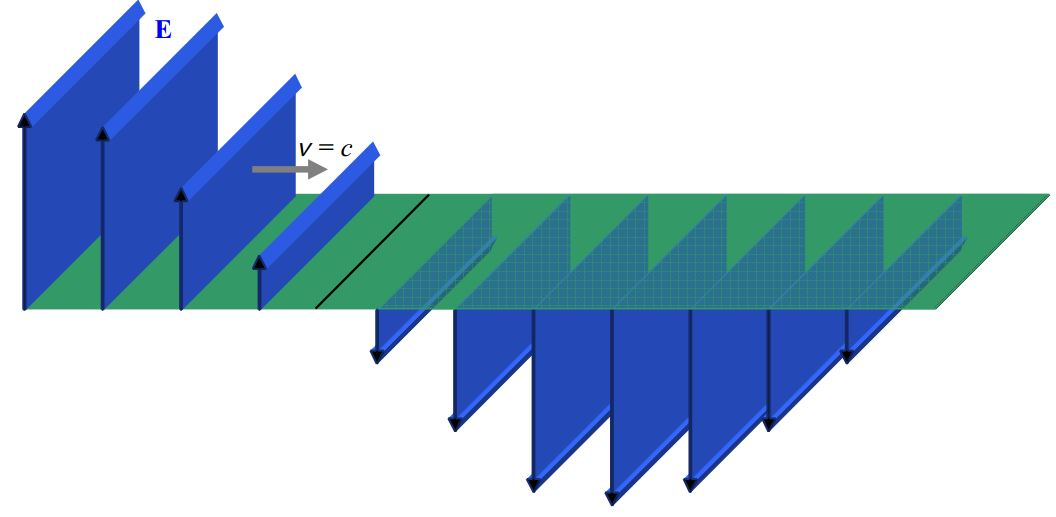This is a simpler diagram with less information which is, perhaps, easier to interpret, but, also, perhaps, easier to misinterpret. It may for instance, be more readily apparent to you that what we are depicting (as we were in the other diagram) is just $$\frac{3}{4}$$ of a wavelength of the wave. On the other hand, the diagram is more abstract—the length of the electric field vectors does not represent extent through space, but rather, the magnitude of the electric field at the tail of the arrow. As mentioned, the set of locations of the tails of the arrows, namely the horizontal plane, is the only place the diagram is giving information. It is generally assumed that the electric field has the same pattern for some distance above and below the plane on which it is specified in the diagram. Note the absence of the magnetic field. It is up to the reader to know that; as part of the light, there is a magnetic field wherever there is an electric field, and that, the greater the electric field, the greater the magnetic field, and that the magnetic field is perpendicular both to the direction in which the light is going, and to the electric field. (Recall that the direction of the magnetic field is such that the vector $$\vec{E}\times \vec{B}$$ is in the same direction as the velocity of the wave.)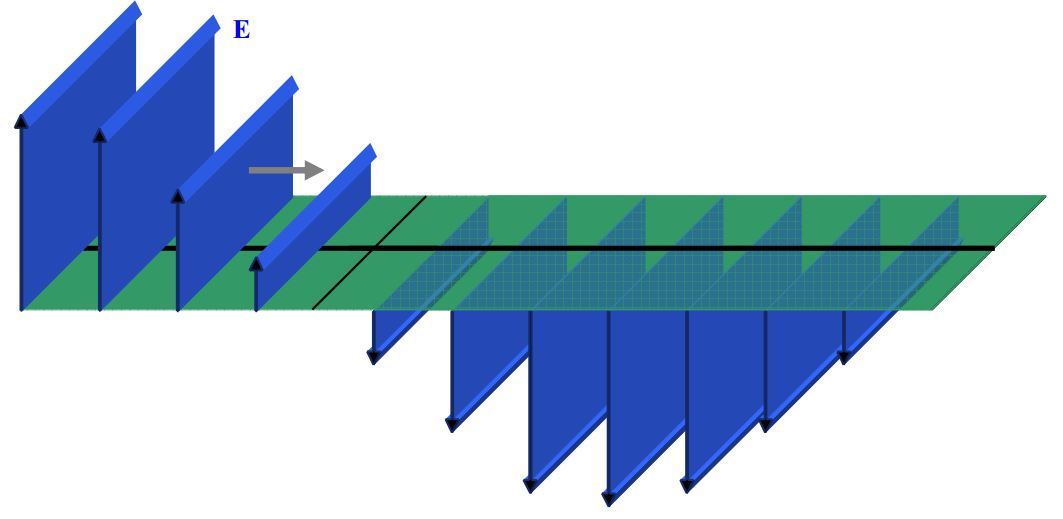An example of an electric field depiction, on a single line along the direction of travel, would be:It is important to keep in mind that the arrows are characterizing the electric field at points on the one line, and, it’s the length of the arrow that indicates the strength of the electric field at the tail of the arrow, not the spacing between arrows.

The simplicity of this field-on-a-line diagram allows for the inclusion of the magnetic field vectors in the same diagram:If one connects the tips of the arrows in this kind of diagram, the meaning of those Electric Field vs. Position sinusoidal curves presented in the last chapter becomes more evident:Huygens’ Principle involves wavefronts. A wavefront is the part of a wave which is at a surface that is everywhere perpendicular to the direction in which the wave is traveling. If such surfaces are planes, the wave is called a plane wave. The kind of wave we have been depicting is a plane wave. The set of fields on any one of the gray “sheets” on in the diagram:is a part of a wavefront. It is customary to focus our attention on wavefronts at which the electric and magnetic fields are a maximum in one direction. The rearmost sheet in the diagram above represents such a wavefront.

In the following diagram, you see black lines on the top of each sheet representing maximum-upward-directed-electric-field wavefronts.And, in the following, each such wavefront is marked with a black dot:A common method of depicting wavefronts corresponds to a view from above, of the preceding electric field sheet diagram which I copy here:Such a wavefront diagram appears as:More commonly, you’ll see more of them packed closer together. The idea is that the wavefronts look like a bird’s eye view of waves in the ocean.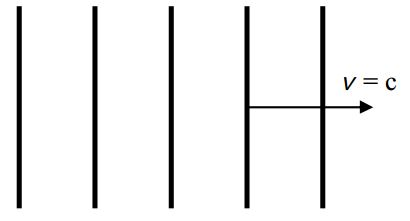Note that the distance between adjacent maximum-field wavefronts, as depicted here, is one wavelength.

At this point we are ready to use the Huygens’s Principle idea, the notion of a wavefront, and our understanding of the way physicists depict wavefronts diagrammatically, to explore the phenomenon of two slit interference.

# Two-Slit Interference

When you shine plane-wave visible light through a mask with two parallel slits cut into it, onto a screen, under certain circumstances (which we shall discuss) you see, on the screen, an extended pattern of bright and dark bands where you might expect to see two bright lines surrounded by darkness which we might call the shadow of the mask.

I use the kind of double slit mask that students make in a double-slit laboratory exercise to convey what we mean by a mask with a double slit in it. We obtain rectangular pieces of thin plate glass of about the size depicted in the following diagram:Prior to the laboratory session, we spray paint each mask with flat black spray paint which is given ample time to dry. Each student is given a razor-blade knife, a metal ruler, and a painted piece of glass that looks like this:The students use the ruler and knife to etch two parallel lines in the paint. After they are done their masks looks like this:The double slit is illuminated with light from a laser. The laser beam hits the mask “head on”. More specifically, the direction in which the light is traveling is at right angles to the surface of the mask. Such light is said to be normally incident upon the mask. (Recall that the word “normal” means “at right angles to.” Make sure you know what we mean by the statement that light is normally incident upon a mask with a double slit in it.)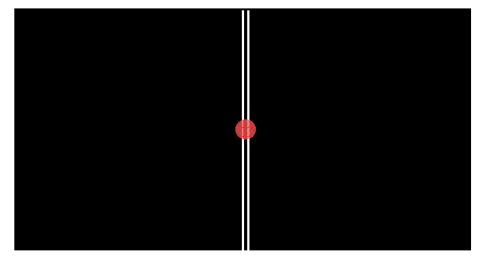On a white paper screen behind the mask, aligned the same way the mask is, the students see a pattern of bright and dark bands distributed horizontally over the face of the screen. The bright bands are typically referred to as fringes. In cases (unlike the laboratory exercise under discussion) where the width of each fringe is small compared to its height, the fringes are often referred to as lines.

Huygens’ Principle helps us understand this phenomenon. The phenomenon is an interference phenomenon. Aside from the need for the slits to be close enough together for the beam to “hit” both slits at the same time, the slits, for reasons that will soon be clear, must be close to each other. In analyzing the phenomenon here, I am going to “zoom in” on the small piece of the mask outlined in the following diagram: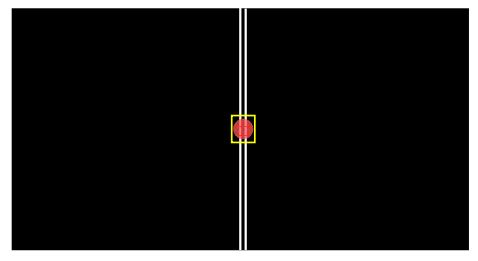I’m going to call the direction in which the light is traveling, on its way to the mask (which is into the page in the diagram above) the forward direction. Now, I am going to depict the entire situation as viewed from above from above.Treating the interface of each slit as a point source in accord with Huygens’ Principle, and representing the intersections of the spherical wavefronts with the plane of the page, we have:The line and circle segments representing the wavefronts correspond to points in space where, at the instant in question, the electric field is maximum in one particular direction. While the direction can be any direction perpendicular to the direction in which the waves are traveling, to make the discussion easier, let’s assume that the electric field oscillations are vertical (into and out of the page) and that the wavefronts represent points in space at which, at the instant in question, the electric field is maximum upward. At those points in space forward of the source, the electric field due to each point-like source varies periodically from upward and maximum (call it $$E_{max}$$), to zero, to downward and maximum ($$-E_{max}$$), back to zero, and back to maximum upward ($$E_{max}$$), with a frequency that we call the frequency of the light waves. At those points in space forward of the source, the total electric field is the sum of the contribution to the electric field from the left slit and the contribution to the electric field from the right slit. Thus, for the instant depicted in the diagram, where the wavefronts cross, we have both contributions at a maximum in one and the same direction. So the total electric field at such points is, at the instant in question, twice that due to either source. As time elapses, the electric field varies at such points, from $$2E_{max}$$ to 0 to $$-2E_{max}$$ to $$0$$, and back to $$2E_{max}$$; repeatedly. The intensity of the light at such a position is $$4$$ times what the intensity due to either slit alone would be. The superposition of the time-varying electric fields is called interference, and when the various contributions add together to form an electric field that varies with an amplitude that is bigger than the electric field due to any individual contributor, we call the interference constructive.

Note that the points where the wavefronts cross lie on, or very near, straight lines extending out from the midpoint between the slits:The big deal is, that at every point on those lines (except “too” close to the slits) the electric field is oscillating with an amplitude of $$2E_{max}$$. If we were to let time continually elapse, you would see those crossing points continually being created near the slits, moving onto the lines and outward along the lines toward the screen. At every point on the lines (except “too” close to the slits), the interference is always constructive at every instant in time. For instance, for the same instant depicted in the diagram above, consider the electric field on the wavefronts midway between the wavefronts depicted. On these “midway between” wavefronts, the electric field is maximum downward. I am going to redraw the wavefront diagram above, minus the maximum-upward (electric field vector) wavefronts, but with the maximum-downward wavefronts (depicted with dashed lines). Again, this diagram is for the same instant in time characterized by the diagram above. Also, I am going to leave those same straight lines along which the constructive interference is occurring, on the diagram.Note that, indeed, (except for “too close” to the slits), the maxima (wavefront crossing points) for the maximum-downward-electric-field vector wavefronts, occur along the exact same lines that the maximum-upward-electric-field vector wavefronts occurred along. Again, I want to stress that the amplitude of oscillations is maximal ($$2E_{max}$$) at every point on each of those line —at points on the lines other than where the wavefronts are crossing, it is just that the electric field is at different stages of those maximum-amplitude oscillations.

Where the lines consisting of points of maximal constructive interference intersect the screen, we see a bright fringe. How about the dark fringes? Those result at points in space where the electric field from one slit always cancels the electric field from the other slit. We can find such points by depicting the maximum-upward-electric-field wavefronts from one slit at a particular instant, while, on the same diagram, depicting the maximum-downward-electric-field wavefronts from the other slit. Where they cross, we have perfect cancellation, not just for the instant depicted, but, for all time.

Here we show maximum-downward-electric-field wavefronts from the left slit interfering with maximum-upward-electric-field wavefronts from the right slit:Again, except for very close to the slits, the points of intersection of the oppositely-directed electric field wavefronts, the points of destructive interference, lie on lines (not the same lines as before) extending outward from the point on the mask that is midway between the slits:Along these lines, the interference is always completely destructive. Where they intersect the screen, we see dark (unilluminated) fringes.

If we put these lines on which destructive interference always occurs on the diagram with the lines along which constructive interference always occurs,we see that the angles at which destructive interference always occurs look like they are about midway between the angles at which constructive interference always occurs.

Okay, it’s time to get quantitative. It’s probably pretty obvious to you that the pattern depends on the wavelength of the light and the spacing between the slits. What we need to do to put an end to this chapter is to find a mathematical relationship between the angles at which the maxima (bright light) and minima (darkness, a.k.a. zero light) occur. I am going to use the symbol $$\theta$$ to represent the angle that whichever maximum or minimum we are focusing on at the time, occurs. We number the maxima 0, 1, 2, … working out from the middle in both directions as indicated on the diagram above. The minima are labeled 1, 2, 3 (there is no “0” minimum), also working out from the middle. To avoid clutter, I have not labeled the minima in the diagram. If necessary, use those numbers as subscripts on the angle $$\theta$$ to distinguish one angle at which a maximum occurs from another, and, to distinguish one angle at which a minimum occurs from another. If it is not clear from the context, the superscripts MAX and MIN may also be needed. The angle of a particular maximum or minimum to the left is always the same as the angle of the corresponding maximum or minimum to the right, so we don’t need to differentiate between left and right.

The two slits represent a special pair of sources. What’s special about such a pair of sources is that the two sources are exactly in synchronization with each other. That is, for instance, when one is producing a maximum-upward electric field vector, so is the other. We say that the two sources are in phase with each other. Another thing that is special about the sources is that, because we consider each slit to be the same size as the other, and the light from the source to be of one and the same intensity across the face of the beam, the amplitude of the oscillations is the same for each. This is why we get total cancellation along the lines on which the minima occur. By inspection of the diagram, it is clear that, to get to any point on the line in the straight-ahead direction, the distance that the light from one slit travels is the same as the distance that light from the other slit travels. As such, at any point along the center line (the line extending from the midpoint between the two slits, straight ahead) whenever a crest (maximum upward electric field) arrives from one slit, a crest arrives from the other slit; and; whenever a trough (maximum downward electric field) arrives from one slit, a trough arrives from the other slit. Thus, on the centerline, the interference is always constructive. So, we have one quantitative result already, a maximum (constructive interference, bright fringe) occurs at $$\theta= 0^{\circ}$$.

Let’s define the center-to-center slit spacing to be $$d$$, and, the wavelength of the incoming plane waves to be $$\lambda$$. We’ll work on finding an expression for the angle $$\theta$$ at which the first maximum occurs, first.Let’s redraw the diagram with a little less clutter to see what’s going on.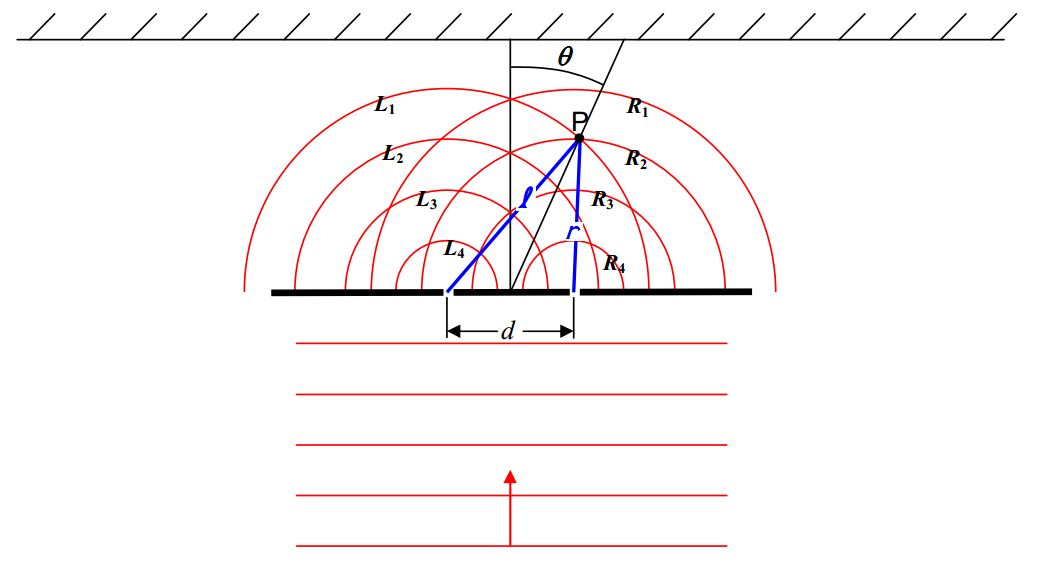I removed some of the spherical wavefronts and labeled the wavefronts from the left slit $$L_1, L_2, L_3,$$ and $$L_4$$ corresponding to the order in which they came from the left slit. Similarly, wavefronts from the right slit are labeled $$R_1, R_2, R_3,$$ and $$R_4$$ corresponding to the order in which they came from the right slit. Furthermore, I have indicated the distance l from the left slit to one point (point $$P$$) on the line of maxima, and, the distance r from the right slit to the same point $$P$$ on the line of maxima.

Look at the diagram and note that, although $$R_1$$ and $$L_1$$ originate from their respective slits at one and the same instant, as do $$R_2$$ and $$L_2$$, it is $$R_2$$ and $$L_1$$ that arrive at point $$P$$ together. By the time it arrives at point $$P$$, $$L_1$$ has been traveling for a greater amount of time than $$R_2$$ has, but, $$L_1$$ arrives at point $$P$$ at the same time as $$R_2$$ does because it ($$L_2$$) has a greater distance to travel. This is the whole key to constructive interference. At any point illuminated by two in-phase sources there will be constructive interference if the point is the same distance from both sources, or, when the distance is different, if that difference is one wavelength, two wavelengths, three wavelengths, or, for that matter, any integer number of wavelengths. If the path difference is an integer number of wavelengths, then, whatever part of the wave is arriving from one source, the same part of the wave will be arriving from the other source. So, for instance, crest arrives with crest, and trough arrives with trough. Defining

$\Delta s= \ell-r$

we have:

$\Delta s=m \lambda\quad (m=0,1,2,...)$

as our condition for constructive interference.

Now I provide the diagram we have been working with, without the wavefronts, so that we can do the geometry needed to relate $$\Delta s$$ to the angle $$\theta$$.First I want to draw a line segment perpendicular to our line of maximum interference, ending on the right slit.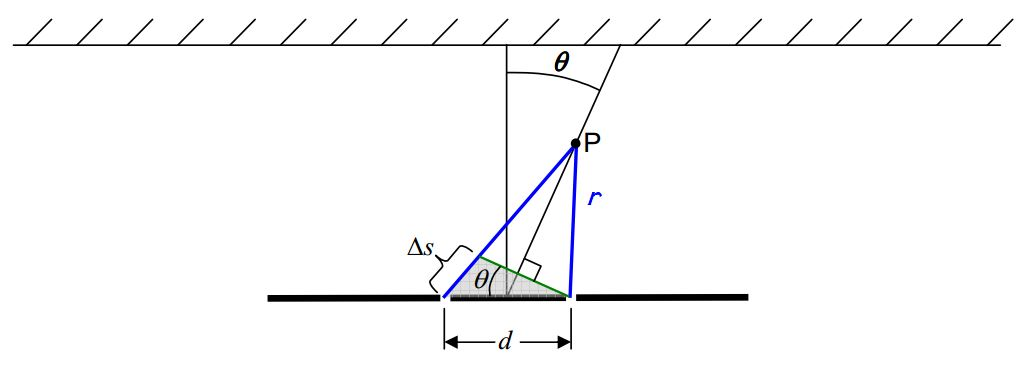The new line segment breaks up the path of length l into a part identical in length to the one of length $$r$$, and a part whose length is the path-length difference $$\Delta s$$. The new line segment also forms a triangle, the one that is shaded in the diagram. Using plane geometry, I have identified the angle labeled $$\theta$$ in the small triangle as the same angle $$\theta$$ that the line of maximal constructive interference makes with the straight-ahead direction. I need to blow up that triangle so that we can analyze it:Okay, now we make an approximation. See angle $$\phi$$ in the shaded triangle? It is approximately equal to $$90^{\circ}$$. Indeed, the farther point $$P$$ is from the slits, the closer $$\phi$$ is to $$90^{\circ}$$. All we need is for the distance from the slits to point $$P$$ to be large compared to the distance between the slits. In practice, this is realized in an actual double-slit experiment. The distance to point $$P$$ is typically thousands to millions of times greater than the distance between the slits. The approximation in question is thus, typically, a fantastic approximation. Treating $$\phi$$ as a right angle makes the shaded triangle a right triangle, meaning that the path difference can be expressed as:

$\Delta s=d \sin \theta$

This is the relation we’ve been looking for. Combining it with the fact that the path difference has to be an integer number of wavelengths for interference maxima we have:

$m\lambda=d\sin\theta\quad (m=0,1,2,...)\label{22-1}$

as the condition for maximum constructive interference.

For perfectly destructive interference, the path difference must be half a wavelength or, half a wavelength more than any integer number of wavelengths. So, for interference minima we have:

$(m+\frac{1}{2})\lambda=d\sin\theta \quad (m=0,1,2,...) \label{22-2}$

The value of the integer m is referred to as the order of interference. Thus, the first maximum to either side of the straight-ahead direction is referred to as the first order maximum, the next one is called the second order maximum, etc.